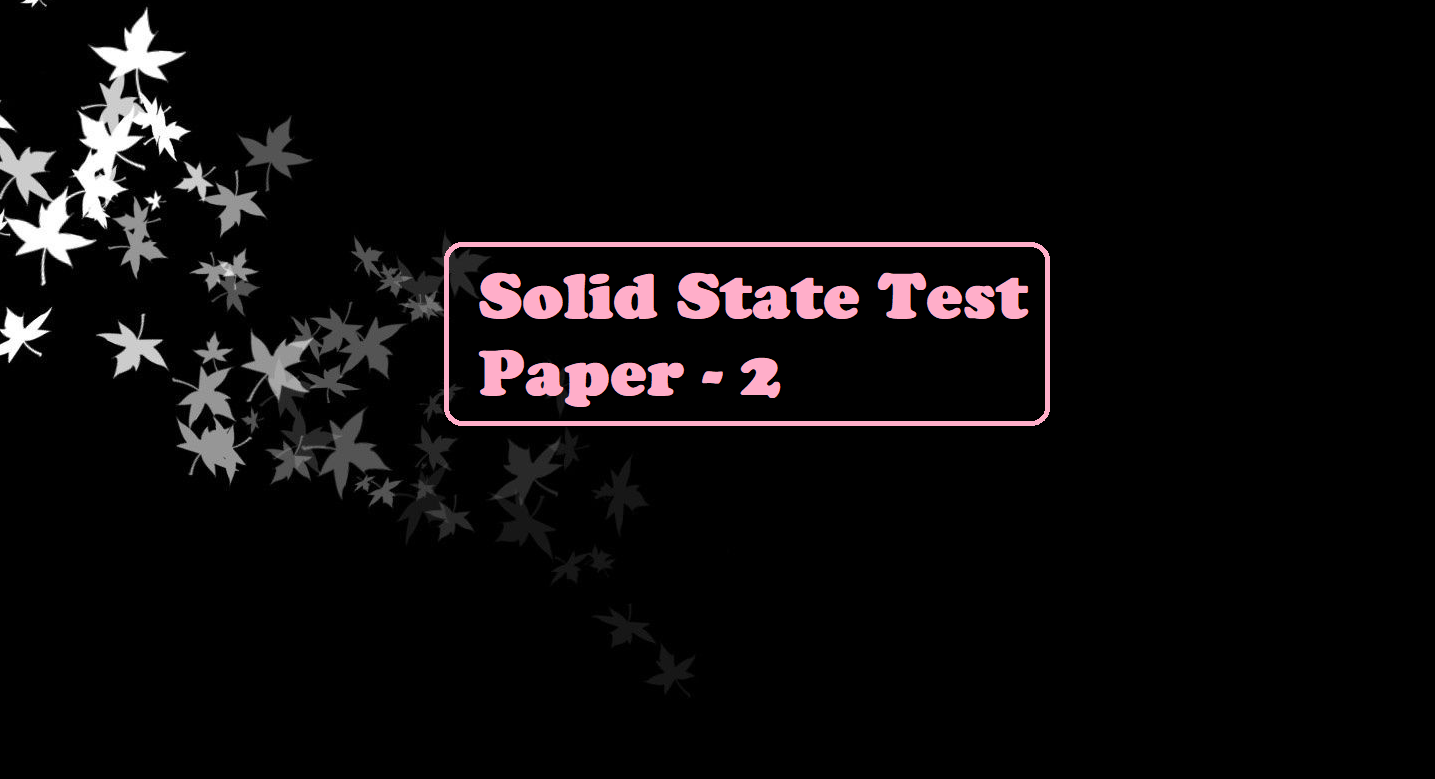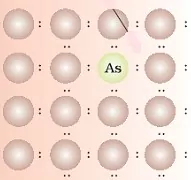# Solid State Test Paper – 2# Solid State Test Paper – 2

Solid State Test Paper – 2

1. The total number of tetrahedral voids in the face-centred unit cell is _____.
(A) 6
(B) 8
(C) 10
(D) 12

2. Solid X is very hard. It is an electrical insulator in solid as well as in molten states and melts at extremely high temperatures. Identify the type of solid X?
(A) Metallic
(B) Covalent
(C) Ionic
(D) Molecular

3. Point defects are present in
(A) Ionic solids
(B) Molecular solids
(C) Amorphous solids
(D) Liquids

4. In which of the following structures coordination number for cations and anions in the packed structure will be the same?
(A) Clion form fcc lattice and Na+ ions occupy all octahedral voids of the unit cell.
(B) Ca2+ ions form fcc lattice and F ions occupy all the eight tetrahedral voids of the unit cell.
(C) O2– ions form fcc lattice and Na+ ions occupy all the eight tetrahedral voids of the unit cell.
(D) S2– ions form fcc lattice and Zn2+ ions go into alternate tetrahedral voids of the unit cell.

5. What is the coordination number in a square close-packed structure in two dimensions?
(A) 2
(B) 3
(C) 4
(D) 6

6. Which stoichiometric defect does not change the density of the crystal?
(A) Frenkel defect
(B) Schottky defect
(C) Interstitial defect
(D) F-centres

7. The edge length of the FCC cell is 508 pm. If the radius of the cation is 110 pm, the radius of the anion is
(a) 110 pm
(b) 220 pm
(c) 285 pm
(d) 144 pm

8. The fraction of the total volume occupied by the atoms present in a simple cube is
(A) π/4
(B) π/6
(C) π/3√2
(D) π/4√2

9. In Zinc blende structure
(A) zinc ions occupy half of the tetrahedral sites
(B) each Zn2- ion is surrounded by six sulphide ions
(C) Each S2- ion is surrounded by six Zn2+ ions
(D) It has an fcc structure

10. Alkali halides do not show Frenkel defect because
(A) Cations and anions have almost equal size
(B) There is a large difference in the size of cations and anions
(C) Cations and anions have a low coordination number
(D) Anions cannot be accommodated in voids

11. The correct order of the packing efficiency in different types of unit cells is ________.
(A) fcc< bcc < simple cubic
(B) fcc> bcc > simple cubic
(C) fcc< bcc > simple cubic
(D) bcc

12. NaCl type crystal (with co-ordination no. 6:6) can be converted into CsCl type crystal (with coordination number 8:8) by applying:
(A) high temperature
(B) high pressure
(C) high temperature and high pressure
(D) low temperature and low pressure

13. Each of the following solids shows the Frenkel defect except:
(A)ZnS
(B) AgBr
(C) AgI
(D) KCl

14. The co-ordination number of cation and anion in Na2O is:
(A) 4 and 6
(B) 6 and 4
(C) 4 and 8
(D) 8 and 4

15. Which compound is added to create an impurity defect in NaCl?
A) AgBr
B) AgCl
C) KI
D) SrCl2

16. The following diagram representsA) Frenkel defects
B) Schottky defects
C) Impurity defects
D) n-type semiconductor

17. In a face-centred lattice of X atoms, Y atoms occupy 1/2 of tetrahedral voids and 1/4 of octahedral voids. What is the possible formula of the compound?
A) X4Y6
B) X4Y5
C) X4Y8
D) X4Y9

18. What type of defect can arise when a solid is heated?
A) Vacancy defect
B) Interstitial defect
C) Metal excess defect
D) Impurity defect

19. The octahedral voids in fcc or ccp are located at
A) Edge centres and along diagonals
B) Edge centres and body centre
C) Along body diagonal and edge centre
D) All the edge centres only

20. Which one of the following is the dimensions of a cubic crystal?
A) a =b ≠ c
B) a = b = c and α = β ≠ γ = 90
C) a = b = c and α = β = γ = 90
D) a ≠ b = c and α = β ≠ γ = 90

Solid State Test Paper – 2

21. Find the coordination number of each type of ions in a rock-salt type crystal structure.

22. An element crystallizes if fcc structure 200 g of this element has 4.12 × 1024 atoms. The density of A is 7.2 g/cm3. Calculate the edge length of the unit cell.

23. Metallic gold crystallizes in the face centre cubic lattice. What is the approximate number of unit cells in 2.0 g of gold? The atomic mass of gold is 197.

24. If three atoms P, Q and R crystallize in a cubic solid lattice with P atoms at the corners, Q atoms at the cube centre and R atoms at the centre of the faces of the cube, write the formula of the compound.

25. Zinc oxide is white, but it turns yellow on heating. Explain.

26. A solid has a cubic structure in which X atoms are located at the corners of the cube, Y atoms are at the cube centres and O atoms are at the edge centres. What is the formula of the compound?

27. What is the coordination number of an atom in each of the following types of cubic lattices?
(i) Primitive             (ii) Face centred        (iii) Body centred

28. The unit cube length of LiCl (NaCl structure) is 5.14 Å. Assuming anion contact, calculate the ionic radius for the chloride ion.

29. An alloy of gold and copper crystallizes in a cubic lattice. In the unit cell, gold atoms occupy the corners of the cube whereas copper atoms occupy the face centres of all the faces of the cube. What is the simplest formula for the alloy?

30. The composition of a sample of wurtzite is Fe0.93O1.00. What percentage of the iron is present in the form of Fe (III)?

Solid State Test Paper – 2